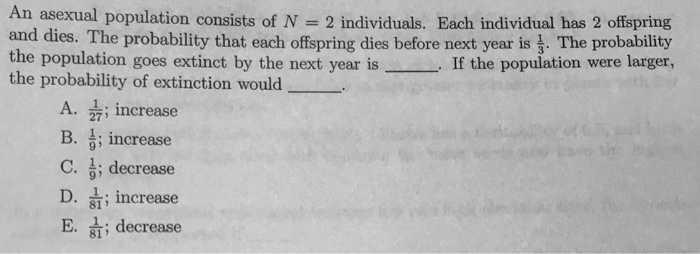# Problem: An asexual population consists of N = 2 individuals. Each individual has 2 offspring and dies. The probability that each offspring dies before next yearis 1/3. The probability the population goes extinct by the next year is _____. If the population were larger, the probability of extinction would _____.A. 1/27; increaseB. 1/9; increaseC. 1/9; decreaseD. 1/81; increaseE. 1/81; decrease

###### FREE Expert Solution

As 2 individuals produce 2 offspring each before dying, there would be 4 individuals in the end. The overall computation of the total probability would require all of these individuals to die.###### Problem Details

An asexual population consists of N = 2 individuals. Each individual has 2 offspring and dies. The probability that each offspring dies before next yearis 1/3. The probability the population goes extinct by the next year is _____. If the population were larger, the probability of extinction would _____.

A. 1/27; increase

B. 1/9; increase

C. 1/9; decrease

D. 1/81; increase

E. 1/81; decrease